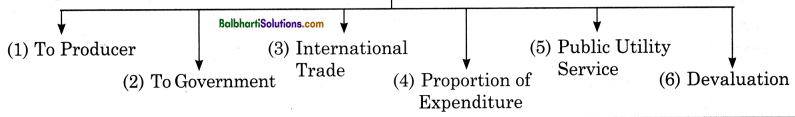By going through these Maharashtra State Board Class 12 Economics Notes Chapter 3B Elasticity of Demand students can recall all the concepts quickly.

## Maharashtra State Board Class 12 Economics Notes Chapter 3B Elasticity of Demand

Definition: According to P. A. Samuelson, “Price elasticity is a concept of measuring how much the quantity demanded responds to changing price.”

Elasticity of demand in fact refers to the degree of responsiveness of the quantity demanded of a commodity to a change in the variable on which demand depends.

Types of Elasticity of Demand:

1. Income Elasticity of Demand
2. Cross Elasticity of Demand
3. Price Elasticity of Demand

→ Income Elasticity of Demand: It refers to the degree of responsiveness of a change in quantity demanded to a change in the income only, other factors including price remaining unchanged.
Ey = $$\frac{Percentage change in Quantity Demanded}{Percentage change in Income}$$

Symbolically, it can be written as follows :
Ey = $$\frac{\frac{\Delta \mathbf{Q}}{\mathrm{Q}}}{\frac{\Delta \mathrm{Y}}{\mathrm{Y}}}$$ = $$\frac{\Delta \mathrm{Q}}{\mathrm{Q}}$$ × $$\frac{\mathrm{Y}}{\Delta \mathrm{Y}}$$

Where,
Q = Original Quantity Demanded
Y = Original Income
ΔQ = Change in Quantity Demanded
Y = Change in income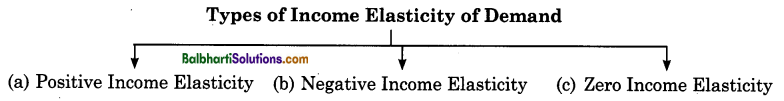→ Positive Income Elasticity: Normal goods which includes necessaries, comforts and luxuries, for which demand increases with increase in income. There is direct relationship between income and quantity demanded. Normal goods have positive income elasticity.

→ Negative Income Elasticity: Inferior goods have a negative income elasticity. As income increases the demand for inferior goods fall. There is an inverse relationship between income and quantity demanded of inferior commodity. Inferior goods are substituted by superior goods.

→ Zero Income Elasticity: When change in income does not have any effect on the demand for a
commodity then the income elasticity is zero for e.g. goods like salt, pins, etc.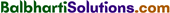Cross Elasticity of Demand: Cross Elasticity of Demand refers to the degree of responsiveness of quantity demanded of a commodity X to a given change in the price of commodity Y. X and Y may be a substitute or complementary goods like tea or coffee or car and petrol.

Cross Elasticity = $$\frac{Percentage change in Quantity demanded of commodity ‘X’}{Percentage change in Price of commodity ‘Y’}$$.

Symbolically, it can be written as follows:
Ec = $$\begin{gathered} \frac{\Delta \mathrm{Q} x}{\mathrm{Q} x} \\ \hline \frac{\Delta \mathrm{P} y}{\mathrm{P} y} \end{gathered}$$ = $$\frac{\Delta \mathrm{Q} x}{\mathrm{Q} x}$$ × $$\frac{\mathrm{P} y}{\Delta \mathrm{P} y}$$

Qx = Original Quantity demanded of commodity ‘X’
Py = Original Price of commodity ‘Y’
ΔQx = Change in Quantity demanded of commodity ‘X’
ΔPy = Change in Price of commodity ‘Y’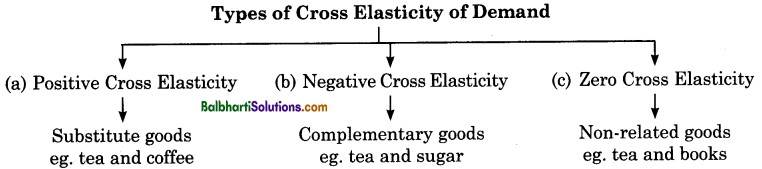Price Elasticity of Demand: Price Elasticity of Demand refers to the degree of responsiveness of quantity demanded of a commodity to the change in its price, other factors remaining constant.

According to Prof. Alfred Marshall, “Price Elasticity of Demand (Ed) is the ratio of proportionat e
change in quantity demanded ola commodity to a given proportionate change in its price.”

Ed = $$\frac{Percentage change in Quantity demanded}{Percentage change in Price}$$
Ed = $$\begin{gathered} \frac{\Delta \mathrm{Q}}{\mathrm{Q}} \\ \hline \frac{\Delta \mathrm{P}}{\mathrm{P}} \end{gathered}$$ = $$\frac{\Delta Q}{Q}$$ × $$\frac{\mathrm{P}}{\Delta \mathrm{P}}$$

ΔQ = Change in quantity demanded (New quantity – Old quantity demanded)
ΔP = Change in Price (New price – Old price)
P & Q = Original price and original quantity demanded.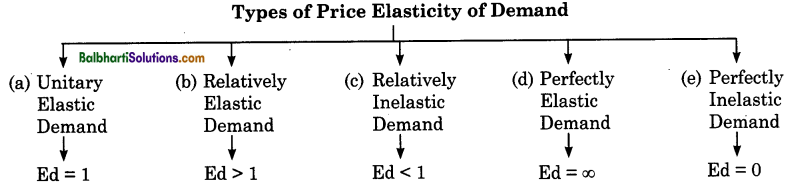(a) Unitary Elastic Demand : (Ed = 1) .
When proportionate or percentage change in quantity demanded is exactly equal to proportionate or percentage change in price, then demand is said to be unitary elastic. For instance a 10% fall in price of a commodity leads to 10% rise in demand of that commodity.

Ed =$$\frac{Percentage change in Quantity demanded}{Percentage change in Price}$$
Ed =$$\frac{10}{10}$$ = 1 Slope of the curve is rectangular hyperbola
∴ Ed = 1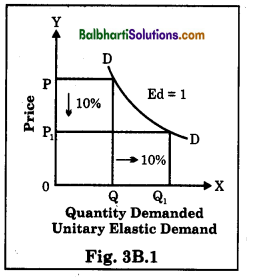(b) Relatively Elastic Demand: (Ed> 1)
When proportionate or percentage change in quantity demanded is more than proportionate or percentage change in price, then demand is said to be relatively elastic demand. For instance a 5% fall in price of a commodity leads to 10% rise in demand of that commodity.
Ed= $$\frac{Percentage change in Quantity demanded}{Percentage change in Price}$$
Ed = $$\frac{10}{5}$$ = 2
i.e. Ed> 1
Slope of the curve is flatter.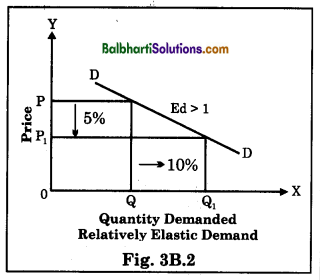(c) Relatively Inelastic Demand : (Ed < 1) When proportionate or percentage change in quantity demanded is less than proportionate or percentage change in price, then demand is said to be relatively inelastic demand.

For instance a 10% fall in price of a commodity leads to 5% rise in demand of that commodity. . Ed = $$\frac{Percentage change in Quantity demanded}{Percentage change in Price}$$ Ed = $$\frac{5 \%}{10 \%}=\frac{1}{2}$$ = 0.5 i.e. e>1
Slope of the curve is steeper slope.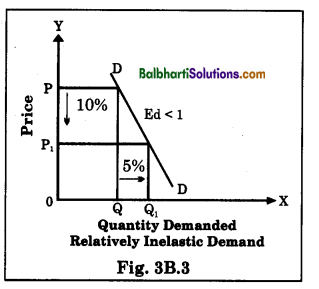(d) Perfectly Elastic Demand or Infinitely Elastic Demand: (Ed =∞)
When a slight change or no change in the price of a commodity Y brings about infinite change in the demand, then it is said to be perfectly elastic demand. For instance, fall in price by 5% may lead to an infinite rise in demand.

Ed = $$\frac{Percentage change in Quantity demanded}{Percentage change in Price}$$
Ed= $$\frac{\text { Any Number }}{0}$$ =∞
e = ∞
Slope of the curve is horizontal slope.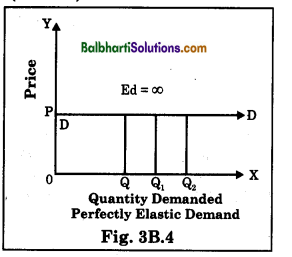(e) Perfectly Inelastic Demand: (Ed = 0)
When a proportionate or percentage change in price brings no effect on quantity demanded of a commodity, then it is said to be perfectly inelastic demand. For instance price falls by 10% or rise by 10%, demand remains constant.

Ed = $$\frac{Percentage change in Quantity demanded}{Percentage change in rice}$$
Ed = $$\frac{0}{10}$$ = 0
e = 0
Slope of the curve is vertical.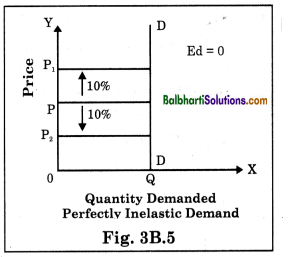Methods of measuring price elasticity of demand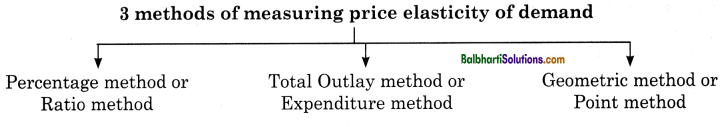Factors influencing Elasticity of Demand:

 Determinants Nature Price Elasticity of Demand Availability of Factors (a) Abundant (b) Few (a) Relatively Elastic (b) Relatively Inelastic Nature of Commodity (a) Necessary (b) Luxury goods (a) Relatively Inelastic (b) Relatively Elastic Habits (a) Habituated (b) Not Habituated (a) Relatively Inelastic (b) Relatively Elastic Time Period (a) Short-run (b) Long run (a) Relatively Inelastic (b) Relatively Elastic Postponement of Consumption (a) Possibility of Postponement (b) Impossible to Postpone (a) Relatively Elastic (b) Relatively Inelastic Number of uses of a commodity (a) Several (b) Specific (a) Relatively Elastic (b) Relatively Inelastic

Importance / Significance of the Concept of Elasticity of Demand: